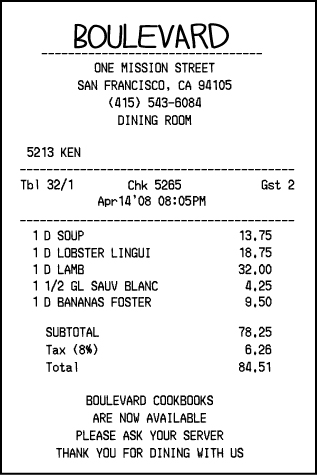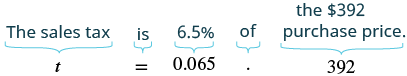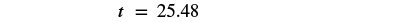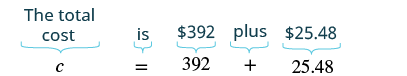## Solving Sales Tax and Commission Applications

### Learning Outcomes

• Solve sales tax applications
• Solve sales commission applications

Sales tax and sales commission are applications of percent in our everyday lives. To solve these types of applications, we will follow the same strategy we used in the section on decimal operations. We show it again here for easy reference.

### Solve an application

1. Identify what you are asked to find and choose a variable to represent it.
2. Write a sentence that gives the information to find it.
3. Translate the sentence into an equation.
4. Solve the equation using good algebra techniques.
5. Check the answer in the problem and make sure it makes sense.
6. Write a complete sentence that answers the question.

Remember that whatever the application, once we write the sentence with the given information (Step 2), we can translate it to a percent equation and then solve it.

## Calculating Sales Tax

Do you pay a tax when you shop in your city or state? In many parts of the United States, sales tax is added to the purchase price of an item (see the image below).  The sales tax is determined by computing a percent of the purchase price.

To find the sales tax multiply the purchase price by the sales tax rate. Remember to convert the sales tax rate from a percent to a decimal number. Once the sales tax is calculated, it is added to the purchase price. The result is the total cost—this is what the customer pays.

The sales tax is calculated as a percent of the purchase price.### Sales Tax

The sales tax is a percent of the purchase price.

$\begin{array}{ccc}\hfill \text{Sales Tax}& =& \text{Tax Rate}\cdot \text{Purchase Price}\hfill \\ \hfill \text{Total Cost}& =& \text{Purchase Price}+\text{Sales Tax}\hfill \end{array}$

### example

Cathy bought a bicycle in Washington, where the sales tax rate was $\text{6.5%}$ of the purchase price. What was

ⓐ the sales tax and
ⓑ the total cost of a bicycle if the purchase price of the bicycle was $\text{\392}?$

Solution

 ⓐ Identify what you are asked to find. What is the sales tax? Choose a variable to represent it. Let $t=$ sales tax. Write a sentence that gives the information to find it. The sales tax is $6.5\text{%}$ of the purchase price. Translate into an equation. (Remember to change the percent to a decimal).Simplify.Check: Is this answer reasonable? Yes, because the sales tax amount is less than $10\text{%}$ of the purchase price. Write a complete sentence that answers the question. The sales tax is $\text{\25.48}$.
 ⓑ Identify what you are asked to find. What is the total cost of the bicycle? Choose a variable to represent it. Let $c=$ total cost of bicycle. Write a sentence that gives the information to find it. The total cost is the purchase price plus the sales tax. Translate into an equation.Simplify.Check: Is this answer reasonable? Yes, because the total cost is a little more than the purchase price. Write a complete sentence that answers the question. The total cost of the bicycle is $\text{\417.48}$.

### try it

In the following video we show an example of how to calculate the sales tax on an item given the tax rate. Note that it does not include calculating the total cost of the item after tax.

## Calculating Sales Tax Rate

Below is an example to show how to find the tax rate for an item given the price after tax, and the original price..

### example

Evelyn bought a new smartphone for $\text{\499}$ plus tax. She was surprised when she got the receipt and saw that the tax was $\text{\42.42}$. What was the sales tax rate for this purchase?

### TRY IT

Watch this video to see another example of how to find the tax rate for an item given the price after tax, and the original price.

## Calculating Original Price

Finally, we see how to calculate the original price given the sales tax rate and the total bill with both the original price plus sales tax as a combined amount.

### Example

Evelyn bought some books at the local bookstore. Her total bill was $31.50$, which included  $5\%$ tax. How much did the books cost before tax?

In the following video example, you will be shown how to find a price before tax using the percent equation.

## Sales Commission

Sales people often receive a commission, or percent of total sales, for their sales. Their income may be just the commission they earn, or it may be their commission added to their hourly wages or salary. The commission they earn is calculated as a certain percent of the price of each item they sell. That percent is called the rate of commission.

### Commission

A commission is a percentage of total sales as determined by the rate of commission.

$\text{commission}=\text{rate of commission}\cdot \text{total sales}$

To find the commission on a sale, multiply the rate of commission by the total sales. Just as we did for computing sales tax, remember to first convert the rate of commission from a percent to a decimal.

### example

Helene is a realtor. She receives $\text{3%}$ commission when she sells a house. How much commission will she receive for selling a house that costs $\text{\260,000}?$

Solution

 Identify what you are asked to find. What is the commission? Choose a variable to represent it. Let $c=$ the commission. Write a sentence that gives the information to find it. The commission is $3\text{%}$ of the price. Translate into an equation.Simplify. $c=7800$ Check. Is this answer reasonable? Yes. $1\text{%}$ of $\text{\260,000}$ is $\text{\2,600}$, and $\text{\7,800}$ is three times $\text{\2,600}$. Write a complete sentence that answers the question. Helene will receive a commission of $\text{\7,800}$.

### try it

In this video, we show another example of how to calculate the amount of commission earned given the rate of commission, and the amount of the sale made.

### example

Rikki earned $\text{\87}$ commission when she sold a $\text{\1,450}$ stove. What rate of commission did she get?

## Contribute!

Did you have an idea for improving this content? We’d love your input.